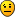## Rolling forefasting evaluation

For questions regarding programming in the EViews programming language.

Moderators: EViews Gareth, EViews Jason, EViews Moderator, EViews Matt

Shwan
Posts: 10
Joined: Thu Dec 21, 2017 7:03 am

### Rolling forefasting evaluation

Hi,
I used the Easther codes of rolling regression for one step ahead forecasting, and I would like to generate rolling forecasting evaluation as well, this is the code that Im using:

' set window size
!window = 729

' set step size
!step = 1

' get size of workfile
!length = @obsrange

' declare equation for estimation
equation eq1

'calculate number of rolls
!nrolls = @floor((!length-!window)/!step)

'matrix to store coefficient estimates
matrix(3,!nrolls) coefmat ' where 3 is the number of coefficients

'series to store forecast estimates
series fcast

' vector to store forecast evaluations
vector (!nrolls) RMSE
vector (!nrolls) THEIL

'*EDITED: catching start and end points
%start = "@first" '@otod(@ifirst(ser))
%end = "@last" '@otod(@ilast(ser))

'variable keeping track of how many rolls we've done
!j=0

' move sample !step obs at a time
for !i = 1 to !length-!window+1-!step step !step
!j=!j+1

' set sample for estimation period
%first = @otod(@dtoo(%start)+!i-1)
%last = @otod(@dtoo(%start)+!i+!window-2)
smpl {%first} {%last}

' estimate equation - where the equation is P =c(1) + c(2)*P1(-1)

' store coefficients
colplace(coefmat,eq1.@coefs,!j)

%1pers = @otod(@dtoo(%start)+!i+!window-1) 'start point
%1pere = @otod(@dtoo(%start)+!i+!window) 'end point
'*EDITED
if @dtoo(%end) < @dtoo(%1pere) then 'check whether the forecast end point is greater than the workfile end point
exitloop
endif

' set smpl for forecasting period
smpl {%1pers} {%1pere}

eq1.forecast(f=na) price_nordf

' set sampl to obtain the 1th period observation
smpl {%1pere} {%1pere}

' Forecast evaluation
!RMSE = @rmse(price, pricef)
!THEIL = @theil(price, pricef)

' store forecasts
fcast = price_nordf
next

smpl @all
show coefmat
show fcast.line

d(noerr) price_nordf

The problem is that these codes dont generate the vector of RMSE and THEIL for rolling evaluation of forecasts, can someone please help me where is the problem in my codes?

Thanks
Shwan

Shwan
Posts: 10
Joined: Thu Dec 21, 2017 7:03 am

### Re: Rolling forefasting evaluation

No answer?Ogi
Posts: 1
Joined: Mon Jun 07, 2021 6:46 am

### Re: Rolling forefasting evaluation

I'm not too confident, but I think I can point out two things.

First, you store the forecasts in "price_nordf", not in "pricef".
Second, the "!RMSE"(!THEIL) is just a new control variable; it is not related to the predefined vector, "RMSE"(THEIL).

So my suggestion is;

RMSE(!j, 1) = @rmse(price, price_nordf)
THEIL(!j, 1) = @theil(price, price_nordf)